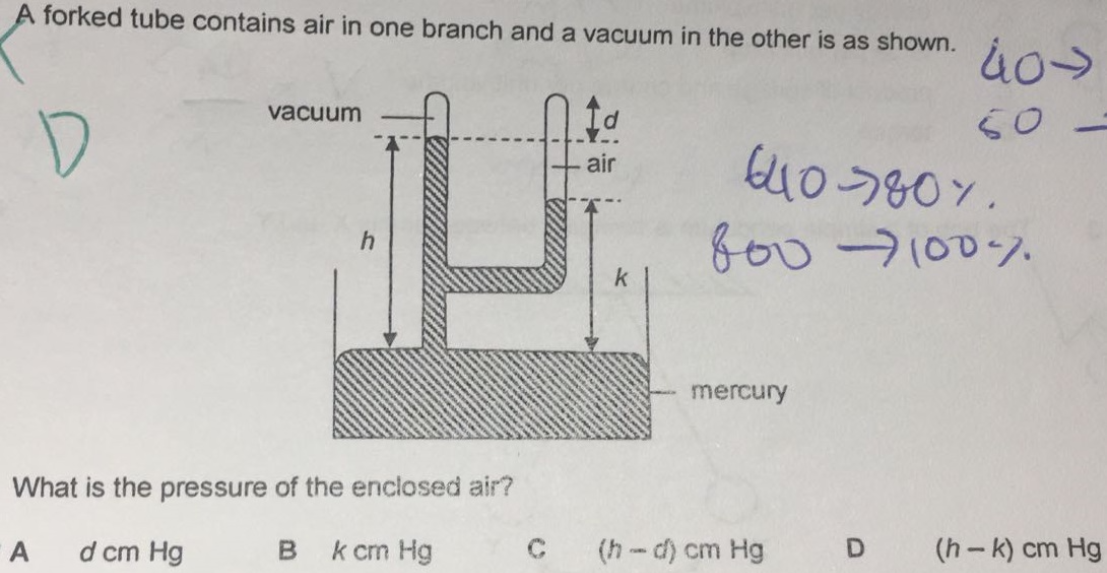# QuestionNeed help with this question.

Concept
Any two points at the same height in a liquid have the same pressure.

Thus, the pressure at height k in the right column, P-right-k, will be equal to the pressure at height k in the left column, P-left-k. In short,
P-right-k = P-left-k —- (1)

Left column
The pressure at the left column is caused by the mercury above. Thus,
P-left-k = (h-k) cmHg —- (2)

Right column
Substituting (2) in (1), we get
P-right-k = (h-k) cmHg

Since the pressure at the surface of the right mercury column is due to enclosed air, the enclosed air pressure = (h-k) cmHg//

0 Replies 1 Like ✔Accepted Answer